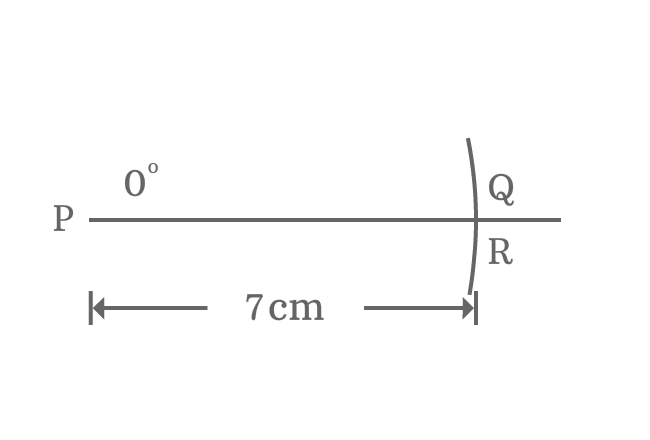# Properties of Right triangle when its angle equals to 0°

## Properties

There are three fundamental properties of a right triangle when its angle is zero degrees.

1. The length of opposite side is zero.
2. The lengths of adjacent side and hypotenuse are equal.
3. The angle of right angled triangle is zero and the other two angles are right angles.

### Theorem$\Delta MON$ is a right triangle and it contains an angle of $0^°$. According to Pythagorean Theorem, the sum of three interior angles of a triangle is $180^°$ geometrically. An angle is $0^°$ and other angle is $90^°$ in the case of right triangle $MON$. So, the third interior angle should be $90^°$.

$\angle MON + \angle ONM + \angle NMO = 180^°$
$\implies 0^° + \angle ONM + 90^° = 180^°$
$\implies \angle ONM = 180^° – 90^°$
$\,\,\, \therefore \,\,\,\,\,\, \angle ONM = 90^°$

Theoretically, it is proved that two angles are right angles when the angle of a right angled triangle is $0^°$.

Assume, the length of adjacent side of this triangle is denoted by $d$.

Zero angle is possible geometrically in a right triangle when its length of opposite side is equal to zero.

$Length \, of \, Opposite \, side$ $\,=\,$ $0$

$\implies$ $MN$ $\,=\,$ $0$

Due to zero length of opposite side, the length of hypotenuse is absolutely equal to the length of hypotenuse. So, remember this property when angle of right triangle is zero.

$\,\,\, \therefore \,\,\,\,\,\,$ $Length \, of \, Adjacent \, side$ $\,=\,$ $Length \, of \, Hypotenuse$ $\,=\,$ $d$

### Proof

A right triangle is required to construct with zero angle for proving all its properties geometrically.1. Draw a horizontal line from point $\small P$ in a plane.
2. Identify zero angle from point $\small P$ and draw zero angle line from point $\small P$ but it appears on horizontal line.
3. Set compass to a length (for example $7 \, cm$) by a ruler. Now, draw an arc on zero angle line and they are intersected at point $\small Q$.
4. It’s not possible to draw a perpendicular line from point $\small Q$ to horizontal line because they both lie on same line. So, assume a perpendicular line is drawn from point $\small Q$ to horizontal line and it intersects the horizontal line at point $\small R$.

A right triangle ($\Delta RPQ$) is constructed with zero degrees angle. Now, it is time to study the properties of right angled triangle when its angle is zero.1. $\small \overline{QR}$ is opposite side (or perpendicular). There is no distance between points $\small Q$ and $\small R$. So, the length of opposite side is zero.
2. $\small \overline{PQ}$ is hypotenuse. It is $7 \, cm$ in this case.
3. $\small \overline{PR}$ is adjacent side (or base). Due to zero length of opposite side, the length of adjacent side is equal to length of hypotenuse exactly. So, the length of adjacent side $\small \overline{PR}$ is also $7 \, cm$.

Therefore, it is proved that the length of opposite side is zero and the length of adjacent side is equal to length of hypotenuse when angle of right triangle is zero.

1. There is no angle between sides $\small \overline{PR}$ and $\small \overline{PQ}$. So, the angle of right triangle is zero. It means $\small \angle RPQ = 0^°$.
2. $\small \overline{QR}$ is a perpendicular line to side $\small \overline{PR}$ at point $\small R$. So, $\small \angle PRQ = 90^°$, which means second angle of right triangle is a right angle.
3. The length of perpendicular line ($\small \overline{QR}$) (known as opposite side) is zero. The hypotenuse and adjacent side are appeared in horizontal line position. Therefore, $\small \angle PQR = 90^°$. In other words, the third angle of right triangle is also a right angle.

Email subscription
Math Doubts is a best place to learn mathematics and from basics to advanced scientific level for students, teachers and researchers. Know more
Follow us on Social Media
###### Math Problems

Learn how to solve easy to difficult mathematics problems of all topics in various methods with step by step process and also maths questions for practising.

Learn more